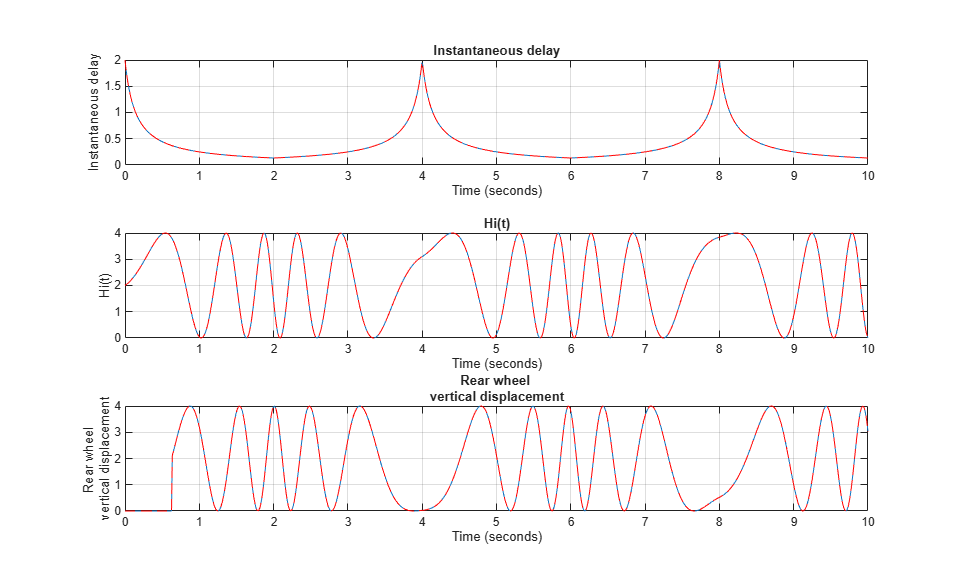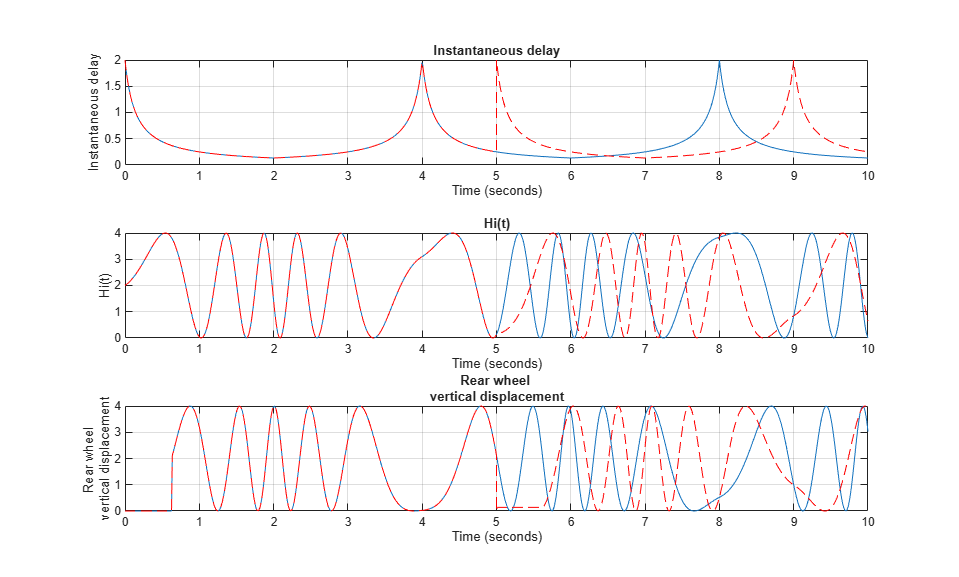# Specify Initial State for Simulation

This example shows the difference between specifying the initial state for a simulation using final states saved with and without the model operating point. Specifying initial states for a simulation can save time when you run a set of simulations. For example, suppose you need to simulate several scenarios for a system that has an initialization phase. If the initialization phase is the same for all scenarios, you can simulate the initialization phase once, save the operating point, and use that operating point as the initial state for all the simulations.

When you want to continue a simulation from a given state, use the model operating point. A model operating point contains complete information about the state of the model and simulation, including the simulation time for the snapshot and the state of the solver and execution engine. When you use a model operating point as the initial state for a simulation, the simulation results match results from a simulation without an initial state, and you do not need to specify the start time for the model. When you specify initial states without the operating point, the simulation results can differ from an equivalent simulation that does not run from an initial state.

For more information about the difference between final states with and without the operating point, see Save Block States and Simulation Operating Points.

### Open and Simulate Model

Open the model `slexVariableTransportDelay`, which models the relationship between the vertical displacement of the front and rear wheels for a car. For more information about the model, see Simulating Systems with Variable Transport Delay Phenomena.

```modelName = "slexVariableTransportDelay"; open_system(modelName) ```This example uses a model with the Variable Transport Delay block to illustrate a key difference between saving final states and saving final states with the operating point. Some blocks, including the Variable Transport Delay block, use information to compute output values that is not included in the logged block state. This information is included in the `Simulink.op.ModelOperatingPoint` object created when you save final states with the final operating point for the model.

Create a `Simulink.SimulationInput` object to specify model parameters to use in the baseline simulation. Configure the model to simulate from `0` to `10` seconds. Use the `OutputTimes` parameter to ensure that the model computes output values for simulation times of `0`, `5`, and `10`.

```siminBaseline = Simulink.SimulationInput(modelName); siminBaseline = setModelParameter(siminBaseline,'StartTime','0','StopTime','10',... 'OutputOption','AdditionalOutputTimes','OutputTimes','[0 5 10]'); ```

Simulate the model to create baseline results.

```baseline = sim(siminBaseline); ```

### Specify Initial State for Simulation Using Model Operating Point

To see how the model operating point allows you to resume a simulation, simulate the model for `5` seconds and save the final operating point. Then, simulate the model again from `5` seconds to `10` seconds using the operating point as the initial state for the model.

Create a `SimulationInput` object to specify model parameters to use in the first simulation. Configure the model to simulate from `0` seconds to `5` seconds, and save the final states with the final operating point.

```siminOP1 = Simulink.SimulationInput(modelName); siminOP1 = setModelParameter(siminOP1,'StartTime','0','StopTime','5',... 'SaveFinalState','on','SaveOperatingPoint','on'); ```

Simulate the model for the first five seconds.

```op1 = sim(siminOP1); ```

Create a `SimulationInput` object to specify model parameters for the second part of the simulation. When you use an operating point as the initial state for a simulation, do not specify the start time for the simulation. The simulation runs from the time saved in the `snapshotTime` property of the `Simulink.op.ModelOperatingPoint` object that contains the operating point data.

```siminOP2 = Simulink.SimulationInput(modelName); siminOP2 = setModelParameter(siminOP2,'StopTime','10'); ```

Specify the initial states for the second portion of the simulation as the operating point saved from the first part of the simulation. By default, the operating point is stored in a variable called `xFinal` and returned as part of the single simulation output as a field in a `Simulink.SimulationOutput` object.

```siminOP2 = setInitialState(siminOP2,op1.xFinal); ```

Simulate the model from five seconds to ten seconds, using the final states without the operating point as the initial state.

```op2 = sim(siminOP2); ```

Plot the results from the interrupted simulation that was resumed using the operating point alongside the results from the baseline simulation.

Get the `Simulink.SimulationData.Dataset` object that contains the signal data logged from the baseline simulation. The results are returned as a single `Simulink.SimulationOutput` object that contains the `Dataset` object with signal logging data in the variable `logsout`.

```baselineResults = baseline.logsout; ```

Combine the logged signal data from the two segments of the interrupted simulation. First, create a copy of the logged signal data that will contain the combined simulation results.

```opResults = op1.logsout; ```

In a `for` loop, access the `timeseries` objects that contain the time and signal data for each logged signal, and use the `append` function to concatenate the data in time. The `Dataset` object with the logged signal data contains a `Simulink.SimulationData.Signal` object for each signal. The `Values` property of the `Signal` object is a `timeseries` object that contains the data. This example uses curly braces (`{}`) to access the `Signal` objects in the `Dataset` objects by index.

```for idx = 1:numElements(op1.logsout) ts1 = op1.logsout{idx}.Values; ts2 = op2.logsout{idx}.Values; opResults{idx}.Values = append(ts1,ts2); end ```

Plot the data on a 3-by-1 MATLAB® figure using a `for` loop to iterate through the elements of the `Dataset` object. This example uses curly braces (`{}`) to access the elements of the `Dataset` objects by index.

```fig2 = figure(2); fig2.Name = "Simulation Resumed Using Operating Point"; for idx=1:numElements(baselineResults) ax = subplot(3,1,idx); plot(baselineResults{idx}.Values) hold on plot(opResults{idx}.Values,'r--') hold off grid on ax.Title.String = baselineResults{idx}.Values.Name; end ```The results from the interrupted simulation that was resumed using the operating point match the results from the uninterrupted simulation.

### Specify Initial State for Simulation Using Final States

To see how using final states without the model operating point can affect simulation results, simulate the model for 5 seconds and save the final states without the operating point. Then, simulate the model again from 5 seconds to 10 seconds, using the final states as the initial state for the simulation.

Create a `SimulationInput` object to specify model parameters to use in the first simulation. Configure the model to simulate from `0` seconds to `5` seconds and save the final states without the operating point.

```siminStates1 = Simulink.SimulationInput(modelName); siminStates1 = setModelParameter(siminStates1,'StartTime','0','StopTime','5',... 'SaveFinalState','on'); ```

Simulate the model for the first five seconds.

```finalStates1 = sim(siminStates1); ```

Create a `SimulationInput` object to specify model parameters for the second part of the simulation. Configure the model to simulate from `5` seconds to `10` seconds. When you use final states without the operating point as the initial state for a simulation, the simulation time is not restored from the final states data. You must specify the start time for the simulation.

```siminStates2 = Simulink.SimulationInput(modelName); siminStates2 = setModelParameter(siminStates2,'StartTime','5','StopTime','10'); ```

Specify the initial states for the second portion of the simulation as the final states saved from the first part of the simulation. By default, final states are stored in a variable called `xFinal` and returned as part of the single simulation output as a field in a `Simulink.SimulationOutput` object.

```siminStates2 = setInitialState(siminStates2,finalStates1.xFinal); ```

Simulate the model from five seconds to ten seconds. Use the final states without the operating point as the initial state.

```finalStates2 = sim(siminStates2); ```

Plot the results for all ten seconds of simulation time side by side to see how resuming the interrupted simulation using final states affects the simulation results.

Combine the logged signal data from the two segments of the interrupted simulation. First, create a copy of the logged signal data that will contain the combined simulation results.

```finalStatesResults = finalStates1.logsout; ```

In a `for` loop, access the `timeseries` objects that contain the time and signal data for each logged signal, and use the `append` function to concatenate the data in time. The `Dataset` object with the logged signal data contains a `Simulink.SimulationData.Signal` object for each signal. The `Values` property of the `Signal` object is a `timeseries` object that contains the data. This example uses curly braces (`{}`) to access the `Signal` objects in the `Dataset` objects by index.

```for idx = 1:numElements(finalStates1.logsout) ts1 = finalStates1.logsout{idx}.Values; ts2 = finalStates2.logsout{idx}.Values; finalStatesResults{idx}.Values = append(ts1,ts2); end ```

Plot the data on a 3-by-1 MATLAB figure using a `for` loop to iterate through the elements of the `Dataset` object. This example uses curly braces (`{}`) to access the elements of the `Dataset` objects by index.

```fig1 = figure(1); fig1.Name = "Simulation Resumed Using Final States Without Operating Point"; for idx=1:numElements(baselineResults) ax = subplot(3,1,idx); plot(baselineResults{idx}.Values) hold on plot(finalStatesResults{idx}.Values,'r--') hold off grid on ax.Title.String = baselineResults{idx}.Values.Name; end ```The simulation results diverge in the interrupted simulation after the simulation is resumed using the final states without operating point information. For this model, the final states alone do not provide enough information to continue the simulation as though it was never interrupted.